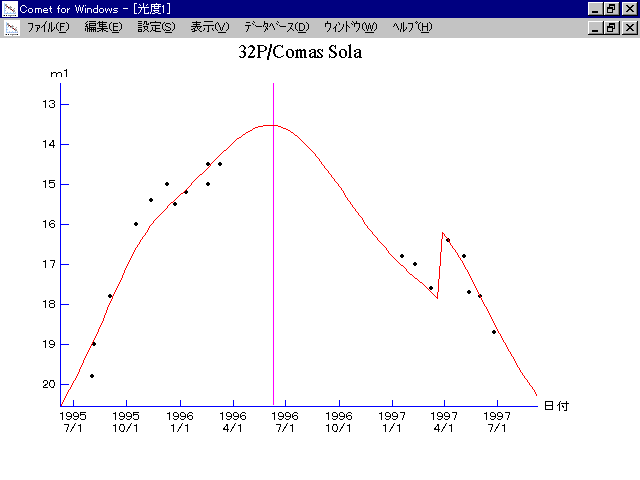# \$B%3%^%9!&%=%iWB@1(B

32P/Comas Sola (1996)###\$B50F;MWAG(B

```   The following improved orbital elements, by Kenji Muraoka, are from
178 observations  1968 to 1997, including 9 Planets, Moon and 5 minor
planets perturbations and non-gravitational effect of style II.
The mean residual is  +/- 0.97 arc seconds.

Epoch  =  1996 June  6.0  TT       JDT = 2450240.5
T  =  1996 June 10.47594       +/- 0.00132 (m.e.) TT
Peri. =   45.76303                +/- 0.00038
Node  =   60.87253                +/- 0.00018   (2000.0)
Incl. =   12.91634                +/- 0.00004
q  =    1.8463610              +/- 0.0000025 AU
e  =    0.5677912              +/- 0.0000006
a  =    4.2719188              +/- 0.0000020 AU
n  =    0.11162709             +/- 0.00000008
P  =    8.829                  +/- 0.0000061  years
A1  =   +0.971                  +/- 0.059
A2  =   -0.11277                +/- 0.00108
```

###\$B@1?^(B1995\$BG/(B 8\$B7n(B 1\$BF|!A(B1996\$BG/(B 7\$B7n(B26\$BF|(B1996\$BG/(B 7\$B7n(B26\$BF|!A(B1997\$BG/(B 5\$B7n(B22\$BF|(B

###\$B8wEYJQ2=(B

```        m1 = 5.3 + 5 log\$B&\$(B + 22.5 log r  [   ,290]  (              \$B!A(B1997\$BG/(B 3\$B7n(B27\$BF|(B)
m1 = 3.5 + 5 log\$B&\$(B + 22.5 log r  [290,   ]  (1997\$BG/(B 3\$B7n(B27\$BF|!A(B              )
```##### \$B50F;MWAG\$OB<2,7r<#;a\$N7W;;\$K\$h\$k\$b\$N\$G\$9!#(B \$B@1?^\$O(B StellaNavigator Ver.2.0 for Windows (\$B%"%9%H%m%"!<%D(B \$BJTCx(B / \$B%"%9%-!<=PHG6I4)(B) \$B\$G:n@.\$7\$?\$b\$N\$G\$9!#(B \$B8wEY%0%i%U\$O(BComet for Windows\$B\$G:n@.\$7\$?\$b\$N\$G\$9!#(B